# NCERT Solutions class 12 Chemistry Solutions In text question## myCBSEguide App

CBSE, NCERT, JEE Main, NEET-UG, NDA, Exam Papers, Question Bank, NCERT Solutions, Exemplars, Revision Notes, Free Videos, MCQ Tests & more.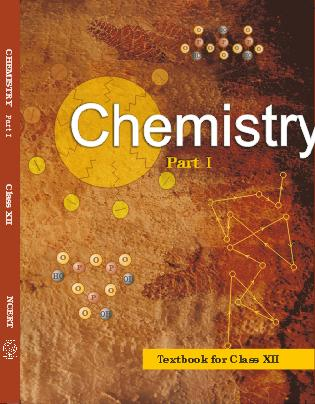## NCERT Class 12 Chemistry Chapter-wise Solutions

•  1 – The Solid State
• 2 – Solutions
• 3 – Electrochemistry
• 4 – Chemical Kinetics
• 5 – Surface Chemistry
• 6 – General Principles and Processes of Isolation of Elements
• 7 – The p-Block Elements
• 8 – The d-and f-Blocks Elements
• 9 – Coordination Compounds
• 10 – Haloalkanes and Haloarenes
• 11 – Alcohols, Phenols and Ethers
• 12 – Aldehydes, Ketones and Carboxylic Acids
• 13 – Amines
• 14 – Biomolecules
• 15 – Polymers
• 16 – Chemistry in Everyday Life

### CHAPTER TWO SOLUTIONS

• 2.1 Types of Solutions
• 2.2 Expressing Concentration of Solutions
• 2.3 Solubility
• 2.4 Vapour Pressure of Liquid Solutions
• 2.5 Ideal and Non-Ideal Solutions
• 2.6 Colligative Properties and Determination of Molar Mass
• 2.7 Abnormal Molar Masses

## NCERT Solutions class 12 Chemistry Solutions In text question

1. Calculate the mass percentage of benzene (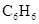) and carbon tetrachloride (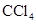) if 22 g of benzene is dissolved in 122 g of carbon tetrachloride.

2. Calculate the mole fraction of benzene in solution containing 30% by mass in carbon tetrachloride.

3. Calculate the molarity of each of the following solutions: (a) 30 g of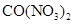.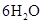in 4.3 L of solution (b) 30 mL of 0.5 M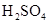diluted to 500 mL.

4. Calculate the mass of urea (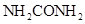) required in making 2.5 kg of 0.25 molal aqueous solution.

5. Calculate (a)molality (b)molarity and (c)mole fraction of KI if the density of 20% (mass/mass) aqueous KI is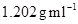.

6.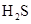, a toxic gas with rotten egg like smell, is used for the qualitative analysis. If the solubility ofin water at STP is 0.195 m, calculate Henry’s law constant.

7. Henry’s law constant for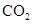in water is 1.67 x 108 Pa at 298 K. Calculate the quantity ofin 500 mL of soda water when packed under 2.5 atmpressure at 298 K.

8. The vapour pressure of pure liquids A and B are 450 and 700 mm Hg respectively, at 350 K. Find out the composition of the liquid mixture if total vapour pressure is 600 mm Hg. Also find the composition of the vapour phase.

9. Vapour pressure of pure water at 298 K is 23.8 mm Hg. 50 g of urea () is dissolved in 850 g of water. Calculate the vapour pressure of water for this solution and its relative lowering.

10. Boiling point of water at 750 mm Hg is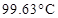. How much sucrose is to be added to 500 g of water such that it boils at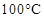. Molal elevation constant for water is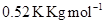.

11. Calculate the mass of ascorbic acid (Vitamin C,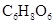) to be dissolved in 75 g of acetic acid to lower its melting point by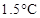.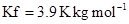.

12. Calculate the osmotic pressure in pascals exerted by a solution prepared by dissolving 1.0 g of polymer of molar mass 185,000 in 450 mL of water at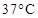.

## NCERT Solutions class 12 Chemistry Solutions In text question

NCERT Solutions Class 12 Chemistry PDF (Download) Free from myCBSEguide app and myCBSEguide website. Ncert solution class 12 Chemistry includes text book solutions from both part 1 and part 2. NCERT Solutions for CBSE Class 12 Chemistry have total 16 chapters. 12 Chemistry NCERT Solutions in PDF for free Download on our website. Ncert Chemistry class 12 solutions PDF and Chemistry ncert class 12 PDF solutions with latest modifications and as per the latest CBSE syllabus are only available in myCBSEguide

To download NCERT Solutions for class 12 Chemistry, Physics, Biology, History, Political Science, Economics, Geography, Computer Science, Home Science, Accountancy, Business Studies and Home Science; do check myCBSEguide app or website. myCBSEguide provides sample papers with solution, test papers for chapter-wise practice, NCERT solutions, NCERT Exemplar solutions, quick revision notes for ready reference, CBSE guess papers and CBSE important question papers. Sample Paper all are made available through the best app for CBSE students and myCBSEguide website.1. Nice